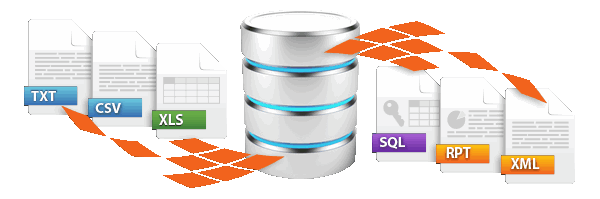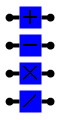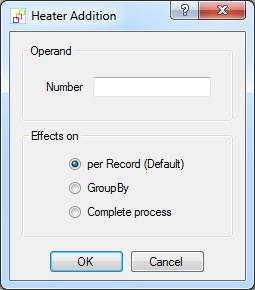Dynamic data import / exportsimply more productive
Up to 100,000 records can be processed with the freeware version!

# Addition - Subtraction - Multiplication - Division HeaterThe Addition, Subtraction, Multiplication and Division Heaters are used to perform their respective arithmetic operations. Each Heater can have any number of inputs, but it makes sense to have at least two inputs. The Addition and Subtraction Heaters can also calculate offsets for dates. Of course, in so doing month and year ends and leap years are taken into account.

Operand (optional)
Using the operand “number” you supply an additional constant to the Heater’s calculation. All values supplied (several input parameters) including the constant value entered here are operated on with the same computation selected.Heater configuration

### Effective on (Addition Heater only)

In the Addition Heater you have the additional option to decide the basis the sum is determined by.

Per record (default): A separate sum is calculated for each record/row processed.

GroupBy (aggregation function): A sum total is calculated for the current grouping.

Complete process: This option generates a sum total for the complete run. e.g. for output on CSV header/footer rows.

### Examples

Please also refer to the general information on the use of Heaters (functions)